An incompressible fluid flows steadily through a cylindrical pipe which has radius 2r at point A and radius r at B further along the flow direction. If the velocity at point A is v, its velocity at point B is

(a) 2v                                (b) v
(c) v/2                               (d) 4v

Concept Questions :-

Equation of continuity
High Yielding Test Series + Question Bank - NEET 2020

Difficulty Level:

A homogeneous solid cylinder of length L(L<H/2) . Cross-sectional area A/5 is immersed such that it floats with its axis vertical at the liquid-liquid interface with length L/4 in the denser liquid as shown in the fig. The lower density liquid is open to atmosphere having pressure ${\mathrm{P}}_{0}$. Then density D of solid is given by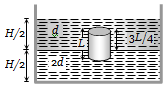(a) $\frac{5}{4}\mathrm{d}$                      (b) $\frac{4}{5}\mathrm{d}$

(c) $\mathrm{d}$                          (d) $\frac{d}{5}$

Concept Questions :-

Archimedes principle
High Yielding Test Series + Question Bank - NEET 2020

Difficulty Level:

A wooden block, with a coin placed on its top, floats in water as shown in fig. The distance l and h are shown there. After some time the coin falls into the water. Then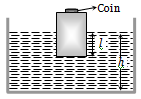(a) l decreases and h increases

(b) l increases and h decreases

(c) Both l and h increase

(d) Both l and h decrease

Concept Questions :-

Archimedes principle
High Yielding Test Series + Question Bank - NEET 2020

Difficulty Level:

A block of ice floats on a liquid of density 1.2 $g/c{m}^{3}$ in a beaker. Then level of liquid when ice completely melts-

(a) Remains same                         (b) Rises
(c) Lowers                                    (d) (a), (b) or (c)

Concept Questions :-

Archimedes principle
High Yielding Test Series + Question Bank - NEET 2020

Difficulty Level:

A vessel of area of cross-section A has liquid to a height H. There is a hole at the bottom of vessel having area of cross-section a. The time taken to decrease the level from ${\mathrm{H}}_{1}$ to ${\mathrm{H}}_{2}$ will be

(a)$\frac{\mathrm{A}}{\mathrm{a}}\sqrt{\frac{2}{\mathrm{g}}}\left[\sqrt{{\mathrm{H}}_{1}}-\sqrt{{\mathrm{H}}_{2}}\right]$              (b) $\sqrt{2\mathrm{gh}}$

(c) $\sqrt{2\mathrm{gh}\left({\mathrm{H}}_{1}-{\mathrm{H}}_{2}\right)}$                      (d) $\frac{\mathrm{A}}{\mathrm{a}}\sqrt{\frac{g}{2}}\left[\sqrt{{\mathrm{H}}_{1}}-\sqrt{{\mathrm{H}}_{2}}\right]$

Concept Questions :-

Bernoulli theorem
High Yielding Test Series + Question Bank - NEET 2020

Difficulty Level:

A lead shot of 1mm diameter falls through a long column of glycerine. The variation of its velocity v with distance covered is represented by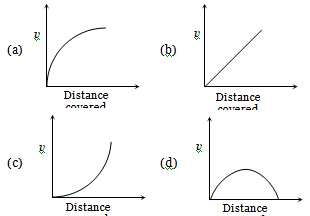Concept Questions :-

Viscosity
High Yielding Test Series + Question Bank - NEET 2020

Difficulty Level:

Water flows through a frictionless duct with a cross-section varying as shown in fig. Pressure p at points along the axis is represented by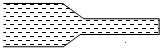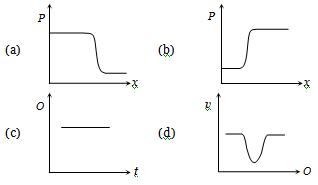Concept Questions :-

Bernoulli theorem
High Yielding Test Series + Question Bank - NEET 2020

Difficulty Level:

From amongst the following curves, which one shows the variation of the velocity v with time t for a small sized spherical body falling vertically in a long column of a viscous liquid -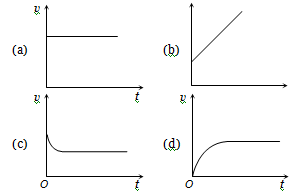Concept Questions :-

Viscosity
High Yielding Test Series + Question Bank - NEET 2020

Difficulty Level:

A liquid does not wet the sides of a solid if the angle of contact is

(a) Zero                                     (b) Obtuse (More than 90°)
(c) Acute (Less than 90°)            (d) 90°

Concept Questions :-

Surface tension
High Yielding Test Series + Question Bank - NEET 2020

Difficulty Level:

The meniscus of mercury in the capillary tube is

(a) Convex            (b) Concave
(c) Plane               (d) Uncertain

Concept Questions :-

Capillary rise